# 如何编写高质量的 JS 函数（1） -- 敲山震虎篇

2019/08/26 14:19

## 一、引言

• 函数(一切皆有可能)

• 函数的命名

• 函数的注释

• 函数的复杂度

• 函数的鲁棒性(防御性编程)

• 函数的入参和出参(返回)

• 如何用函数式编程打通函数的任督二脉

• 如何用设计模式让函数如虎添翼

• 编写对 V8 友好的函数是一种什么 style

• 前端工程师的函数狂想录

## 二、函数(一切皆有可能)

PS: 注意，没有之一。

### 2、函数的执行机制

``````function say() {
let str = 'hello world'
console.log(str)
}``````

#### （1）创建函数

``````let str = 'hello world'
console.log(str)``````

PS: 建议去学习一下数据结构，栈中的一块一块的，我们称为帧。你可以把栈理解中 DOM 树，帧理解为节点，每一帧( 节点 )都有自己的名字和内容。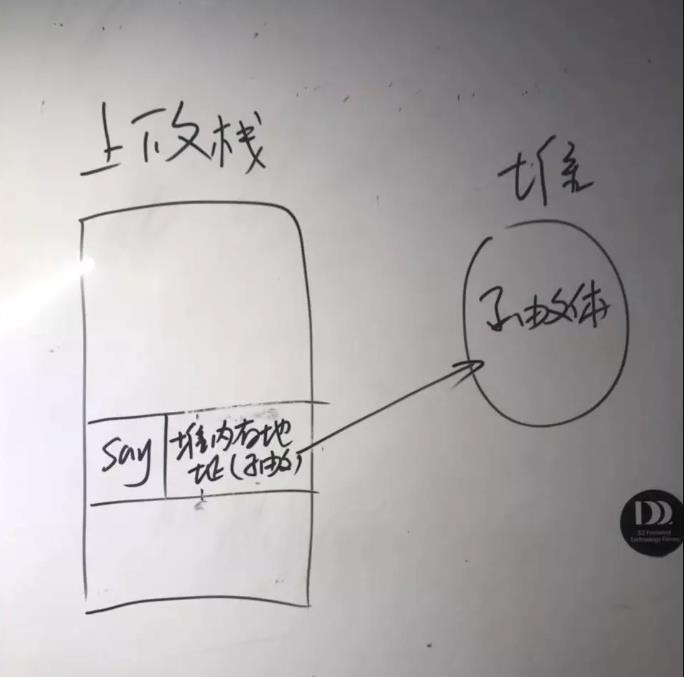#### （2）你真的懂赋值这个操作吗？

``````start：
mov ax, 1
mov bx, 1
end start;``````

PS: 所以如果是值类型，那就是直接把数据，流(移动)到指定内存地址的存储空间中。

#### （4）将字符串变成真正的 JS 代码

``````function f1() {
let b = 1;
function f2() {
cnsole.log(b)
}
return f2
}

let fun = f1()
fun()``````

#### （5）开始执行函数

• 这个供代码执行的环境是什么？

• 这个栈内存是怎么分配出来的？

• 这个栈内存的内部是一种什么样的样子？

## 三、谈谈底层实现

### 1、计算机中最本质的闭包解释

• 第一点：把原有堆内存中存储的字符串变成真正的 JS 代码。
• 第二点：保护该栈内存的私有变量不受外界的干扰。

### 2、栈内存是怎么分配出来？

JS 的栈内存是系统自动分配的，大小固定。如果自动适应的话，那就基本不存在除死循环这种情况之外的栈溢出了。

### 3、这个栈内存的内部是一种什么样的样子？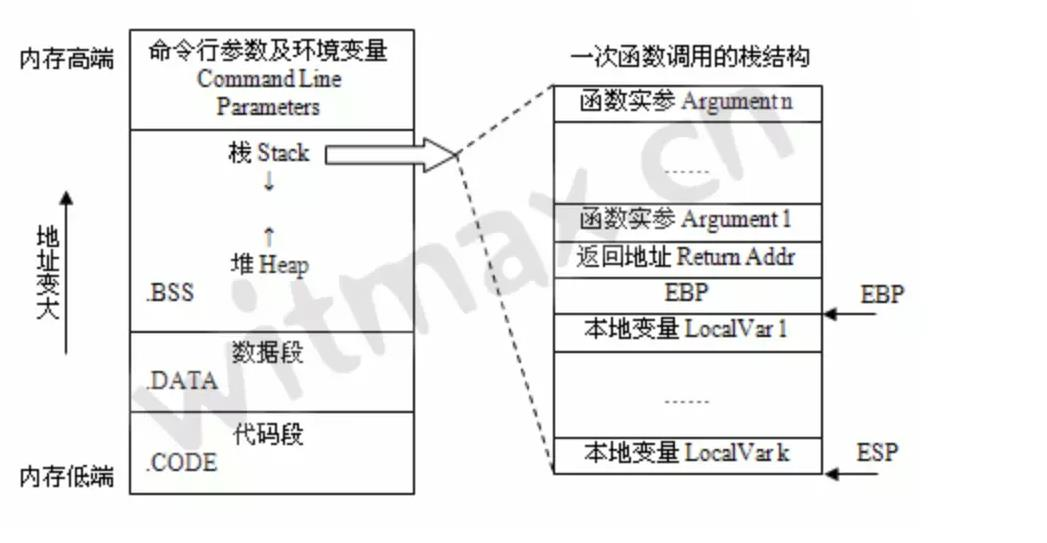``````function f1() {
return 'hello godkun'
}
let result = f1()
f2(result)``````

``````function main() {
say()
// TODO:
say()
}``````

## 四、JS 引擎是如何执行函数

``````//定义一个全局变量 x
var x = 1
function A(y) {
//定义一个局部变量 x
var x = 2
function B(z) {
//定义一个内部函数 B
console.log(x + y + z)
}
//返回函数B的引用
return B
}
//执行A,返回B
var C = A(1)
//执行函数B
C(1)``````

JS 引擎构造的 ESCstack 结构如下：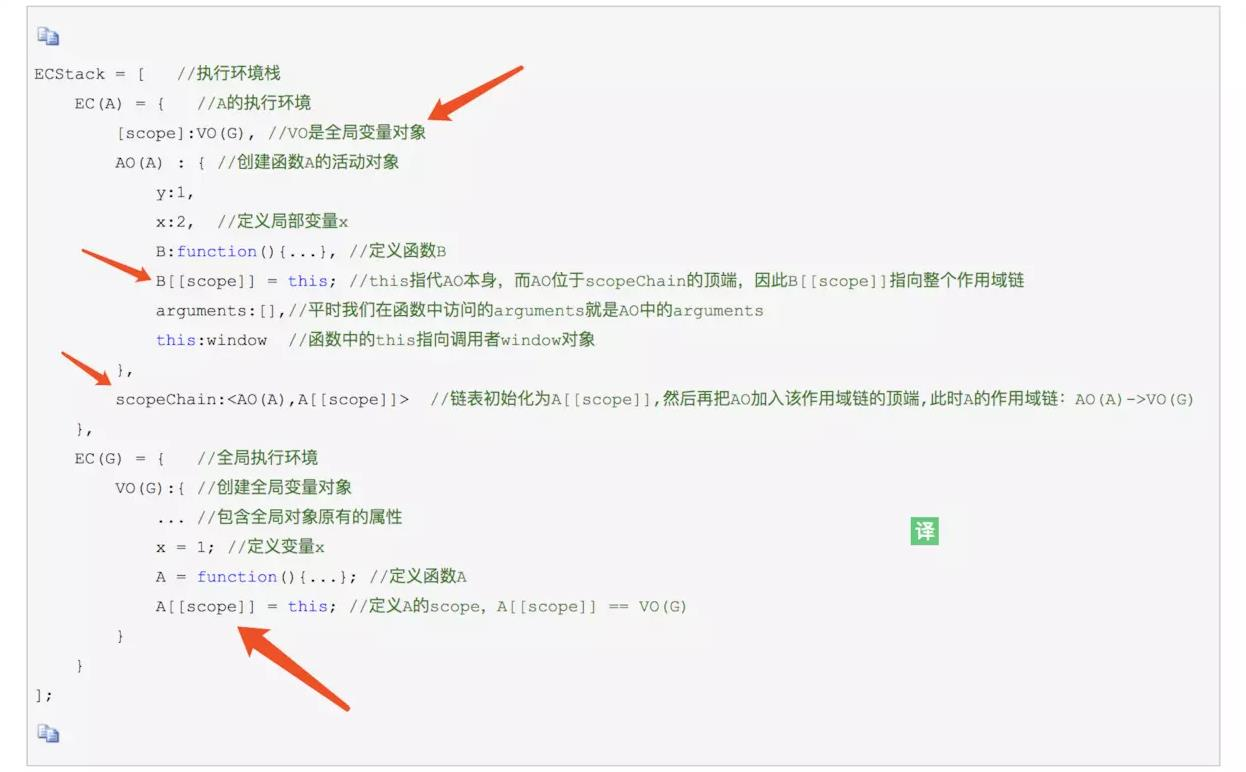JS 引擎构造的 ESCstack 结构如下：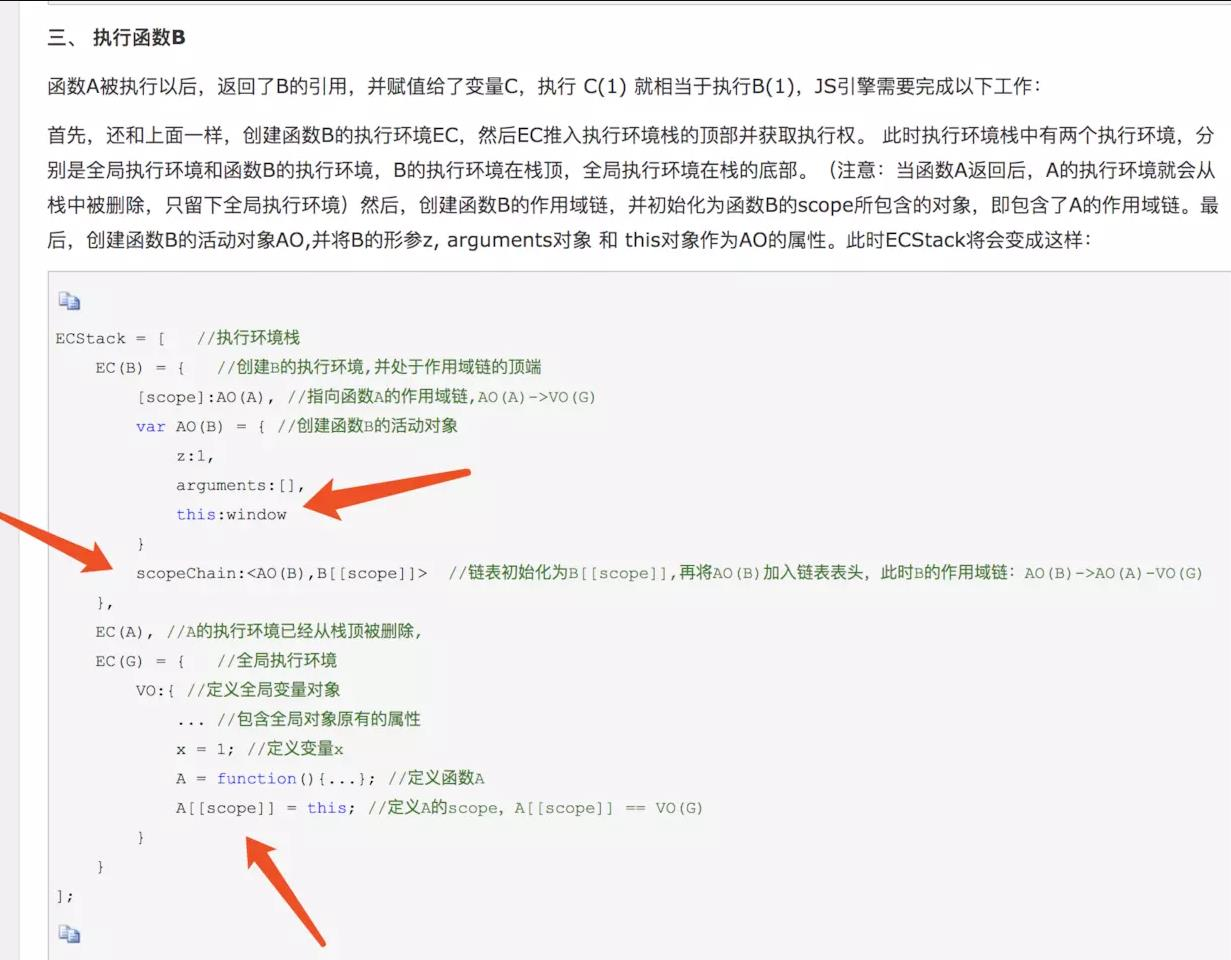### 1、局部变量是如何被保存起来的

``````EC(B) = {
[scope]:AO(A),
var AO(B) = {
z:1,
arguments:[],
this:window
},
scopeChain:<AO(B),B[[scope]]>
}``````

scopeChain 是作用域链，熟悉数据结构的同学肯定知道我函数作用域链本质就是链表，执行哪个函数，那链表就初始化为哪个函数的作用域，然后把当前指向的函数活动对象放到 scopeChain 链表的表头中。

this 为什么在运行时才能确定

this 的指向真相

### 5、作用域的本质是链表中的一个节点

[scope] 指向的是一个函数活动对象，核心点是把这个函数对象当成一个作用域，最好理解成一个链表节点。

PS: B 执行 B 函数时，只有 B 函数有 this 属性，这也就交叉证实了 this 只有在运行时才会存在。

6、作用域链的本质就是链表

## 五、用一道面试题让你更上一层楼(走火入魔)

``````function kun() {
var result = []
for (var i = 0; i < 10; i++) {
result[i] = function() {
return i
}
}
return result
}

let r = kun()
r.forEach(fn => {
console.log('fn',fn())
})``````

``````function kun() {
var result = []
for (var i = 0; i < 10; i++) {
result[i] = (function(n) {
return function() {
return n
}
})(i)
}
return result
}

let r = kun()
r.forEach(fn => {
console.log('fn', fn())
})``````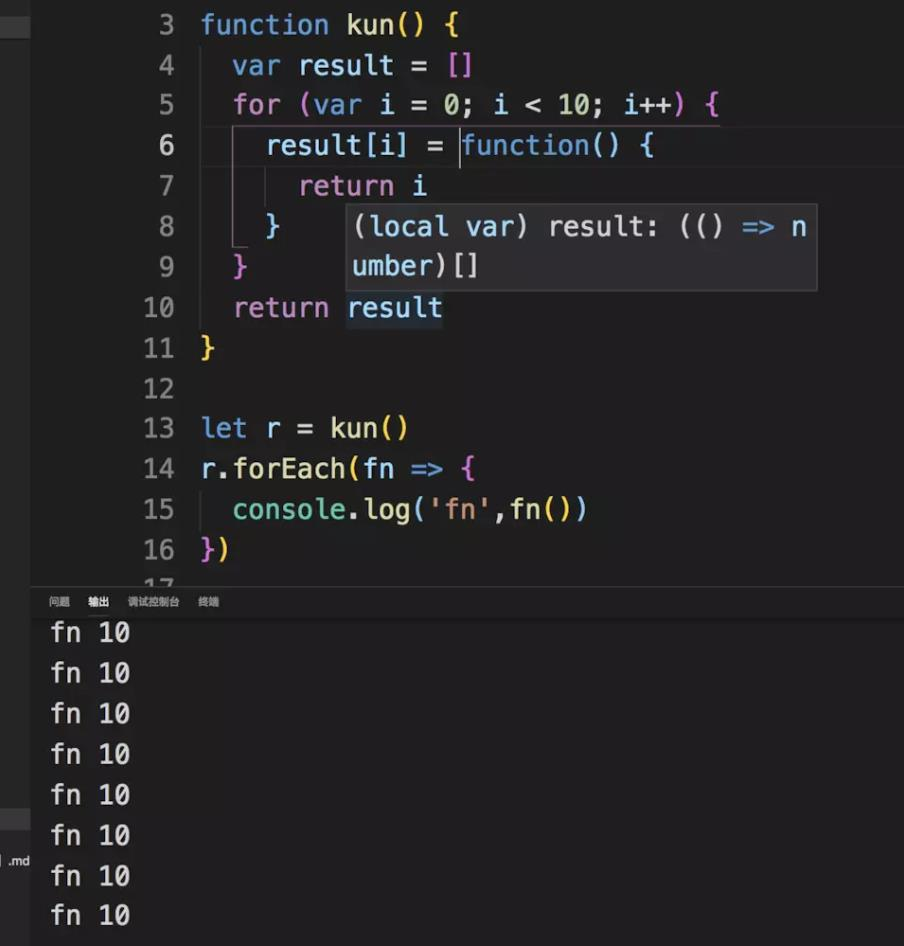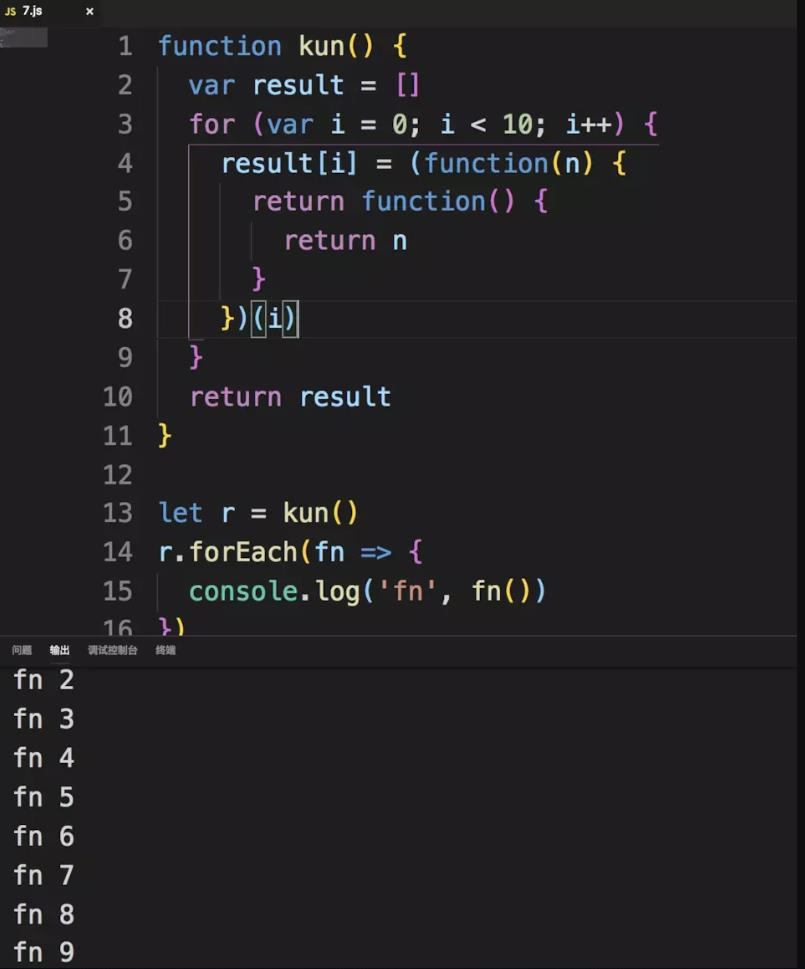• 一部分 coder 只能答到立即调用，闭包。

• 大多数 coder 可以答到作用域相关知识。

• 极少部分 coder (大佬级别) 可以从核心底层原因来分析。

### 1、分析输出10个10

``````function kun() {
var result = []
for (var i = 0; i < 10; i++) {
result[i] = function() {
return i
}
}
return result
}

let r = kun()
r.forEach(fn => {
console.log('fn',fn())
})``````

``````AO(kun):{
i = 10;
kun = function(){...};
kun[[scope]] = this;
}``````

``AO(kun) --> VO(G)``

``AO(result[i]) --> AO(kun) --> VO(G)``

### 2、分析输出0到9

``````function kun() {
var result = []
for (var i = 0; i < 10; i++) {
result[i] = (function(n) {
return function() {
return n
}
})(i)
}
return result
}

let r = kun()
r.forEach(fn => {
console.log('fn', fn())
})``````

``````ECSack = [
EC(kun) = {
[scope]: VO(G)
AO(kun) = {
i: 0,
result = function() {...// return i},
arguments:[],
this: window
},
scopeChain:<AO(kun), kun[[scope]]>
},
// .....
EC(kun) = [
[scope]: VO(G)
AO(kun) = {
i: 9,
result = function() {...// return i},
arguments:[],
this: window
},
scopeChain:<AO(kun), kun[[scope]]>
]
]``````

• result 函数的父执行环境是 EC(kun) ，这个 VO(kun) 里面的 i 值 是 0 。

• result 函数的父执行环境是 EC(kun) ，这个 VO(kun) 里面的 i 值 是 9 。

``AO(result[i]) --> AO(kun) --> VO(G)``

## 七、参考文档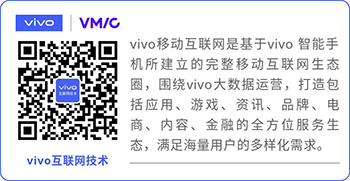15
104 收藏

### 作者的其它热门文章10/14 21:16

2019/10/12 14:28

2019/09/01 11:36

2019/08/27 21:17

ts 会引领时代
2019/09/01 10:13

😊
2019/08/26 17:06

6 评论
104 收藏
15